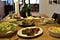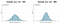# Statistics — Statistical Sampling and Sampling Distributions

Statistical SamplingVerdict Foodservice (2019)
1. Population & Sample

As in this article we are going to talk about statistical sampling, the first thing we should know is the difference between a population and a sample. Suppose we want to investigate the average share of income do households in U.K. spend in restaurants. Our population in this case will be all households currently located in U.K., theoretically speaking, we could knock each door of the households and get the answer. However, in this way, it would be costly and time-consuming to implement and aggregate all the answers. Instead, we could first get a sample of 100 households, then generalize our results from the sample to the population.

We now take a look at the definitions:

‘A population consists of all items of interest for a particular decision or investigation.’

‘A sample is a subset of a population.’

‘Sampling is the foundation of statistical analysis. We use sample data in business analytics applications for many purposes.’ Such as estimating the mean, variance, or proportion of a very large or unknown population, providing values for inputs in decision models and understanding customer satisfaction etc.

2. Sampling Methods

There are many choices of sampling methods exist. In order to draw valid conclusion from our results, we should determine which sampling method to use. Basically, sampling methods can be categorized into two types: probability sampling and non-probability sampling. Probability sampling involves selecting the items using some random procedure, thus, probability sampling allows us to draw valid statistical conclusions about the population. Non-probability sampling involves non-random procedure based on judgement or convenience. The method of sampling we select is crucial for the whole sampling process, below are the lists of some common sampling methods that we usually use.

Sampling Distributions

1. Estimators

An estimator is a statistic used to estimate some fact about the population, in other words, an estimator could be regarded as the rule that creates an estimate. Thus, an estimate is the value of the estimator using a specific sample. Recall the example we used in first section, we want to investigate the average share of income do households in U.K. spend in restaurants. Suppose now we got a sample of 100 households from the population.

Also, we could also use the sample variance to estimate a population variance, and the sample proportion to estimate a population proportion.

2. Sampling Error

When we randomly took a sample from a population, the value of statistics computed by analyzing the sample, i.e. estimates of a population parameters, will differ from the value of statistics computed by analyzing the entire population to some extent. Sampling error occurs because samples are only a subset of the population. Although sampling error could be minimized, it could not be totally avoided.

Another type of error is called non-sampling error, non-sampling error occurs while the sample took could not represent the target population adequately. For example, poor sampling design or targetting the wrong population frame would cause non-sampling errors. There are many types of non-sampling errors and the names used might differ.

‘To draw good conclusions from samples, analysts need to eliminate non-sampling error and understand the nature of the sampling error.’

3. Sampling Distributions

Now we can consider our example, we want to investigate the average share of income do households in U.K. spend in restaurants.

• We set the sample size to 100 households.
• Then we take repeated samples of the size 100 from the population, for each samples we calculate the sample mean and record the result.
• At last, we plot a histogram of the sample means.

Following above steps, we could get the sampling distribution of the sample mean. Thus, we would give a definition to sampling distribution:

‘A sampling distribution is a probability distribution of statistic (such as the mean) that results from selecting an infinite number of random samples of the same size from a population.’

Note that the sampling distributions are theoretical, instead of selecting an infinite number of random samples, we would conduct repeated sampling, and use the central limit theorem to build the sampling distribution.

‘The central limit theorem states that if the sample size is large enough, the sampling distribution of the mean is approximately normally distributed, regardless of the distribution of the population and that the mean of the sampling distribution will be the same as that of the population.

Another key result about the sampling distribution of the mean that statistians found is the standard deviation of the sampling distribution of the mean, also called the standard error of the mean. Here is the function:

From the function, we could see that as n increases, the value of the standard error of the mean will decrease. This suggests that if we take larger sample sizes, we will have less sampling error, in other words, the variance of the sampling distribution goes down. In our example, instead of setting the sample size as 100, we will set the sample size to 300. The conclusion is more intuitional if we plot different sample sizes in graphs.— ‘Estimation’ [PowerPoint presentation] Grigorios Emvalomatis

Reference

Emvalomatis, G. (2020) ‘Estimation’ [PowerPoint presentation]. BU52018: Business Analytics.

McCombes, S. (2019). An introduction to sampling methods. scribbr. https://www.scribbr.com/methodology/sampling-methods/

McLeod, S. A. (2019). Sampling distribution. Simply Psychology. https://www.simplypsychology.org/sampling-distribution.html